login  home  contents  what's new  discussion  bug reports help  links  subscribe  changes  refresh  edit

Computing value of following polynomial was proposed as a benchmark:

fricas
(1) -> p := x^24+32*x^21+9*x^18+34*x^12+34*x^10+45*x^3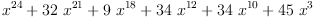(1)
Type: Polynomial(Integer)

Naive solution have unimpressive speed:

fricas
)set messages time on
[eval(p, x, 0.5) for i in 1..1000];
Type: List(Polynomial(Float))
fricas
Time: 0.12 (IN) + 0.09 (EV) + 0.01 (OT) = 0.22 sec

Simple reformulations do not give better results. However, assuming that we need double precision floating point results we can turn p into a compiled function. First we convert polynomial to use doubles as coefficients:

fricas
pf := p::POLY(DoubleFloat)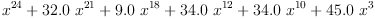(2)
Type: Polynomial(DoubleFloat?)
fricas
Time: 0 sec

Then we define corresponding function:

fricas
function(pf, 'dff, 'x)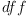(3)
Type: Symbol
fricas
Time: 0.01 (OT) = 0.01 sec

Now try it:

fricas
v := 0.5::SF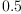(4)
Type: DoubleFloat?
fricas
Time: 0 sec
[dff(v) for i in 1..10000];
fricas
Compiling function dff with type DoubleFloat -> DoubleFloat
Type: List(DoubleFloat?)
fricas
Time: 0.15 (EV) + 0.01 (OT) = 0.16 sec

Now it is much better, but still slow. Main cost is interpreter loop. Try driver function:

fricas
do_it(v, n) == (res : SF := 0; for i in 1..n repeat res := res + dff(v); res)
Type: Void
fricas
Time: 0 sec
do_it(v, 1000000)
fricas
Compiling function do_it with type (DoubleFloat, PositiveInteger)
-> DoubleFloat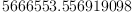(5)
Type: DoubleFloat?
fricas
Time: 0.61 (EV) + 0.01 (OT) = 0.62 sec

This is much better, around half microsecond per evaluation, but still slower than machine arithmetic. Profiling (not shown here) indicated that 99% of time is spent in exponentiation routine. So we would need better formula like Horner rule.

 Subject:   Be Bold !! ( 14 subscribers )